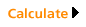HOME MANUFACTURERS PULP PAPER CONVERTERS CHEMICALS MACHINERY CONTROL SYSTEM SERVICE PROVIDERS DISTRIBUTORS/TRADERS CONSULTANTS EMPLOYMENT WASTE MANAGEMENT TESTING REPAIR SCHOOLS ORGANIZATIONS PAPER INFORMATION ABBREVIATION DICTIONARY ASK HARRY PULP &PAPER GRADES PULP &PAPER PROPERTIES WASTE PAPER GRADES PAPER MAKING PROCESS ARTICLES OTHER RESOURCES MAGAZINES CONFERENCES COUNTRY WISE STAT.

CALCULATION OF LENGTH OF PAPER IN A ROLL

Paper Length Calculation when Diameter of Roll & Paper Thickness is Known

If you know the outer diameter of the roll and the core on which paper is wound and the thickness of the paper, you can calculate the length of paper using following equations.

Outer Roll Diameter                            Do
Outer Diameter of Core                      Di
Thickness of Paper                                t

English or US Units (Diameter in Inches and thickness in Point. Please note Point is 1/1000th of an Inch)

Length of Paper in Feet:            1000*3.1426*(Do^2 - Di^2) = 65.47*(Do^2 - Di^2)
(t*12*4)                                        t

Example: Let Outer Diameter of Roll (Do) is 40", Outer Diameter of Core (Di) is 6.5" and thickness is 8 Pt.

Length of Paper in Feet = 65.47*(40*40 - 6.5*6.5)/8 = 12,748 ft

Metric or ISO Units (Diameter in Centimetres and thickness in Micrometres)

Length of Paper in Meter:            1000*3.1426*(Do^2 - Di^2) = 78.56*(Do^2 - Di^2)
(t*10*4)                                        t

Example: Let Outer Diameter of Roll (Do) is 100 cm, Outer Diameter of Core (Di) is 16 cm and thickness is 200 micrometer.

Length of Paper in Meter = 78.56*(100*100 - 16*16)/200 = 3827 m

Open an Excel Sheet to calculate paper length

Please note that these equations assume that there is no air gap between adjacent paper layers hence this formulae should not be used for tissue and some other bulky paper rolls. Actual length may be less than the calculated length.

Paper Length Calculation when Weight of Roll & Paper Basis Weight is Known

If you know the net weight (Total weight - Core weight) of the roll and the Basis weight of the paper, you can calculate the length of paper using following equations.

Net weight of Paper                            M
Width of Roll                                      W
Basis Weight of Paper                          G

Metric or ISO Units (Weight in Kgs, Width in cm  and Basis Weight in g/m2)

Length of Paper in Meter:             100,000*M
W*G

Example: Let Net Weight of Roll (M) is 500 Kgs, Width of Roll (W) is 100 cm and Basis weight or GSM is 100g/m2.

Length of Paper in Meter = 100,000*500/100*100 = 5000 m

English or US Units (Weight in lbs, Width in Inches  and Basis Weight in lbs)

Easiest way is to convert lbs weight in to Basis weight or GSM

To use the Weight Conversion tool, please select a Type of Paper from the pull down menu, then enter a value in pounds and hit calculate. To reverse the calculation simply select "gsm2->lbs" and enter a gsm value.

lbs -> g/m2
g/m2->lbs
 Type of Paper Book Bond Newsprint Cover Index Vellum Tag lbs Grams/Square meterPoint to Paper Weight Conversion (This is true for typical uncoated paper and assume 12 Pt. thick is equivalent to 270 gsm)

 convert pt to gsm to mm

Length of Paper in Meter:             58,590*M
W*G

Example: Let Net Weight of Roll (M) is 1100 lbs, Width of Roll (W) is 40" and Basis weight or GSM is 100g/m2.

Length of Paper in Feet = 58,590*1100/40*100 = 16,112 ft

Open an Excel Sheet to calculate paper length

Various Calculator available to calculate length of paper in a roll

http://www.handymath.com/cgi-bin/rollen.cgi?submit=Entry

Roll Weight Calculation when Diameter of Roll & Paper Thickness is Known

If you know the outer diameter of the roll and the core on which paper is wound and the thickness of the paper and basis weight, you can calculate the weight of paper on roll using following equations.

Outer Roll Diameter                            Do
Outer Diameter of Core                      Di
Width of Roll                                       W
Thickness of Paper                                 t
Basis Weight of paper                            g

English or US Units (Diameter in Inches and thickness in Point. Please note Point is 1/1000th of an Inch)

Weight of Paper in Pound (lb):            0.001117*(Do^2 - Di^2)*W*g
t

Example: Let Outer Diameter of Roll (Do) is 40", Outer Diameter of Core (Di) is 6.5", thickness is 8 Pt., Width of roll is 40" and Basis weight is 100 g/m2

Weight of Paper in Pound = 0.001117*(40*40 - 6.5*6.5)*40*100/8 = 870.2 lbs

Metric or ISO Units (Diameter and width in Centimetres, basis weight in g/m2 and thickness in Micrometres)

Weight of Paper in Kg:             0.0007855*(Do^2 - Di^2)*W*g
t

Example: Let Outer Diameter of Roll (Do) is 100 cm, Outer Diameter of Core (Di) is 16 cm, Width of roll is 100 cm, basis weight is 100  g/m2 and thickness is 200 micrometer.

Weight of Paper in Kg = 0.0007855*(100*100 - 16*16)*100*100/200 = 382.8 Kg

Open an Excel Sheet to calculate paper weight